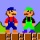Home
IT Knowledge
Inspiration
Languages
EN

# JavaScript - run-length encoding example

7 points
Created by:Zayyan-Todd
560

In this short article, we would like to show how to compress data with Run-length encoding (RLE) in JavaScript.

Run-length encoding (RLE) is a lossless compression method that is widely used when some data elements are repeated one after one in some sequence, e.g. PNG format uses it - useful to save UI screenshots.

## 1. Main compression concept

The RLE output is written as pair of a number of occurrences and occurred characters.

Note: next section shows the RLE concept on letters compression.

Input data:

``AAAAAABBBBBBACCCCCCCCCCCCCDDDAAAAAAAAAAAAA``

Compressed data:

``6A6B1A13C3D13A``

## 2. Letters compression example

Compression results for letters sequence can be written as pairs of a number of letter occurrences and occurred letter - separator, in that case, is not needed.

``````// ONLINE-RUNNER:browser;

function compressText(text) {
var result = '';
if (text.length > 0) {
var count = 1;
var value = text;
for (var i = 1; i < text.length; ++i) {
var entry = text[ i ];
if (entry == value) {
count += 1;
} else {
result += count + '' + value;
count = 1;
value = entry;
}
}
result += count + '' + entry;
}
return result;
}

// Example:

var text = 'AAAAAABBBBBBACCCCCCCCCCCCCDDDAAAAAAAAAAAAA';
var compressedText = compressText(text);

console.log(compressedText);  // 6A6B1A13C3D13A``````

## 3. Coma separated compression example

When we use letters and numbers it is necessary to separate pairs in some way (e.g. `aaaaabbb` is equivalent for `5:a,3b`).

``````// ONLINE-RUNNER:browser;

function compressText(text) {
var result = '';
if (text.length > 0) {
var count = 1;
var value = text[ 0 ];
for (var i = 1; i < text.length; ++i) {
var entry = text[ i ];
if (entry == value) {
count += 1;
} else {
result += count + ':' + value + ',';
count = 1;
value = entry;
}
}
result += count + ':' + entry;
}
return result;
}

// Example:

var text = 'AAAAAA55555ACCCCCCCCCCCCC111AAAAAAAAAAAAA';
var compressedText = compressText(text);

console.log(compressedText);  // 6:A,5:5,1:A,13:C,3:1,13:A``````

## 4. Array compression example

Another way to represent compressed data is to separate result items with comas keeping in mind: 2 items in output represent one unique character input sequence (e.g. `aaaaabbb` is equivalent for `5,a,3,b`).

``````// ONLINE-RUNNER:browser;

function compressArray(array) {
var result = [ ];
if (array.length > 0) {
var count = 1;
var value = array[ 0 ];
for (var i = 1; i < array.length; ++i) {
var entry = array[ i ];
if (entry == value) {
count += 1;
} else {
result.push(count);
result.push(value);
count = 1;
value = entry;
}
}
result.push(count);
result.push(value);
}
return result;
}

// Example:

var array = [
1, 1, 1, 1, 1, 1,                       //  6x 1
6, 6, 6, 6, 6,                          //  5x 6
1,                                      //  1x 1
3, 3, 3, 3, 3, 3, 3, 3, 3, 3, 3, 3, 3,  // 13x 3
8, 8, 8,                                //  3x 8
1, 1, 1, 1, 1, 1, 1, 1, 1, 1, 1, 1, 1   // 13x 1
];
var compressedArray  = compressArray(array);

console.log(compressedArray );  // 6,1,5,6,1,1,14,3,3,8,13,1``````

## References

1. Run-length encoding - Wikipedia